• ## Adding basic vision - Part 2 of Solving Puzlogic puzzle game with Python and OpenCV

In the previous post we looked into building a basic solver of the Puzlogic puzzle. Now let’s take a look at creating the Vision component of the bot.

The vision component needs to be able to:

• Identify the game board, which cells are already filled in, which ones may have pieces placed into them;
• Identify the set of available pieces a level provides;
• Identify values of the available pieces and filled-in game cells.

### Planning

As before, we’ll use `OpenCV` for vision, `mss` for taking screenshots of the window, and `Pillow` (fork of `PIL`) to convert screenshots into OpenCV compatible images. For character recognition of piece values we’ll use `Pytesseract` bindings for `Tesseract`.

We’ll implement `get_game_board()`, `get_cells()` and `get_pieces()` public methods, as well as a way to OCR piece values.

#### Taking screenshots

Let’s start by building an easier way to campture screenshots of the window.

``````import cv2
import numpy as np
import time
import pytesseract
from PIL import Image
from mss import mss
from collections import namedtuple

class ScreenshotSource:
def __init__(self):
self.monitor = {'top': 0, 'left': 0, 'width': 1920, 'height': 1080}
self.screen = mss()
self.image = None

def get(self):
if self.image is None:
self.image = self.refresh()

return self.image
``````

Here I’m hardcoding the monitor’s dimensions to 1920x1080 - the common Full HD screen. It would be better to pass it in as a constructor argument, but for now that’ll do.

`get` method will be used to return the screenshot for further processing. It will be taking the screenshot of the full screen, including OS toolbars, browser window and hopefully game window. Image itself will get cached for improved performance until we actually need to refresh the frame.

``````def refresh(self):
source_image = self.screen.grab(self.monitor)
rgb_image = Image.frombytes('RGB', source_image.size, source_image.rgb)
rgb_image = np.array(rgb_image)
bgr_image = self.convert_rgb_to_bgr(rgb_image)

return bgr_image

def convert_rgb_to_bgr(self, img):
return img[:, :, ::-1]
``````

We take a screenshot with `mss`, convert the binary output to RGB with `PIL`, then convert the RGB (Red, Green, Blue) image into BGR (Blue, Green, Red) color space. This is important, as OpenCV internally works with BGR images.

RGB to BGR conversion works by keeping X and Y coordinates the same (first two arguments), but reversing the order of the third timension, turning RGB values into BGR.

#### Finding game area

Now that we have the screenshot in bot’s memory, we can start by identifying where within the window game area is.

``````class Vision:
def __init__(self, source):
self.source = source

def get_game_board(self):
""" Detects the game window area within a computer screen """

screen_image = self.source.get()
original_screen_image = screen_image.copy()
grayscale = cv2.cvtColor(screen_image, cv2.COLOR_BGR2GRAY)

# Find black background around the game screen
ret, mask = cv2.threshold(grayscale, 1, 255, cv2.THRESH_BINARY)

# Eliminate noise and smaller elements
kernel = cv2.getStructuringElement(cv2.MORPH_CROSS, (3, 3))
dilated = cv2.dilate(binary_grayscale, kernel, iterations=1)

_, contours, _ = cv2.findContours(dilated, cv2.RETR_LIST, cv2.CHAIN_APPROX_SIMPLE)

Board = namedtuple('Board', ['x', 'y', 'w', 'h', 'screen'])

for contour in contours:
# get rectangle bounding contour
[x, y, w, h] = cv2.boundingRect(contour)

# Discard small pieces, we're looking for a game window roughly 800x600
if w < 700 or h < 500 or w > 800:
continue

cropped = original_screen_image[y:y+h, x:x+w]

return Board(x, y, w, h, cropped)

return False
``````

The basic idea is to turn the image into binary grayscale for easier management (pure white or pure black, turns the 3D array into 2D one), run a dilation kernel on the full image to eliminate smaller elements and noise (as that increases the number of contours), and then look for a rectangular contour that is close in size to the target game area (800x600 px).

1. We start with the original image.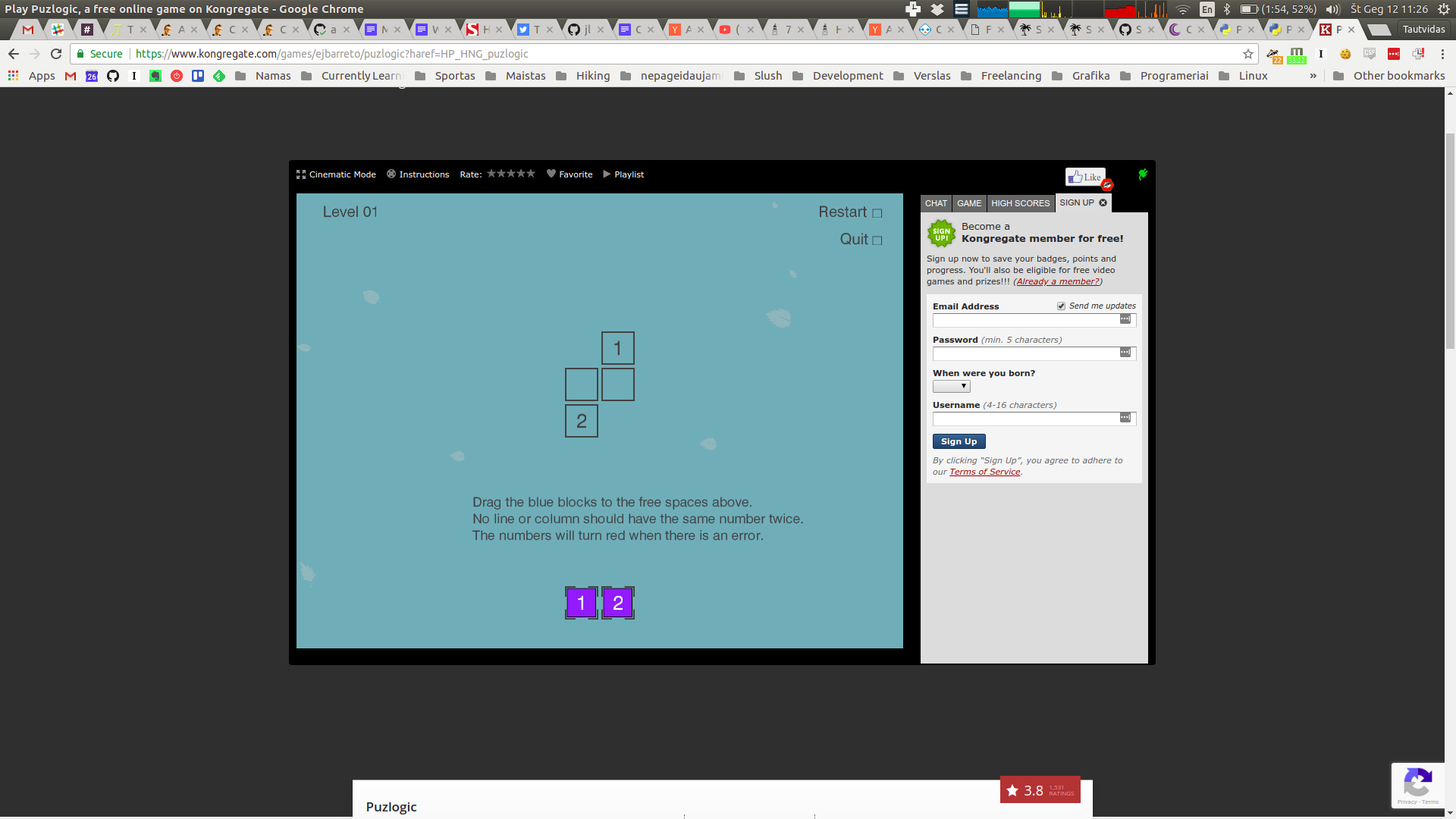2. Turn the image to grayscale color space.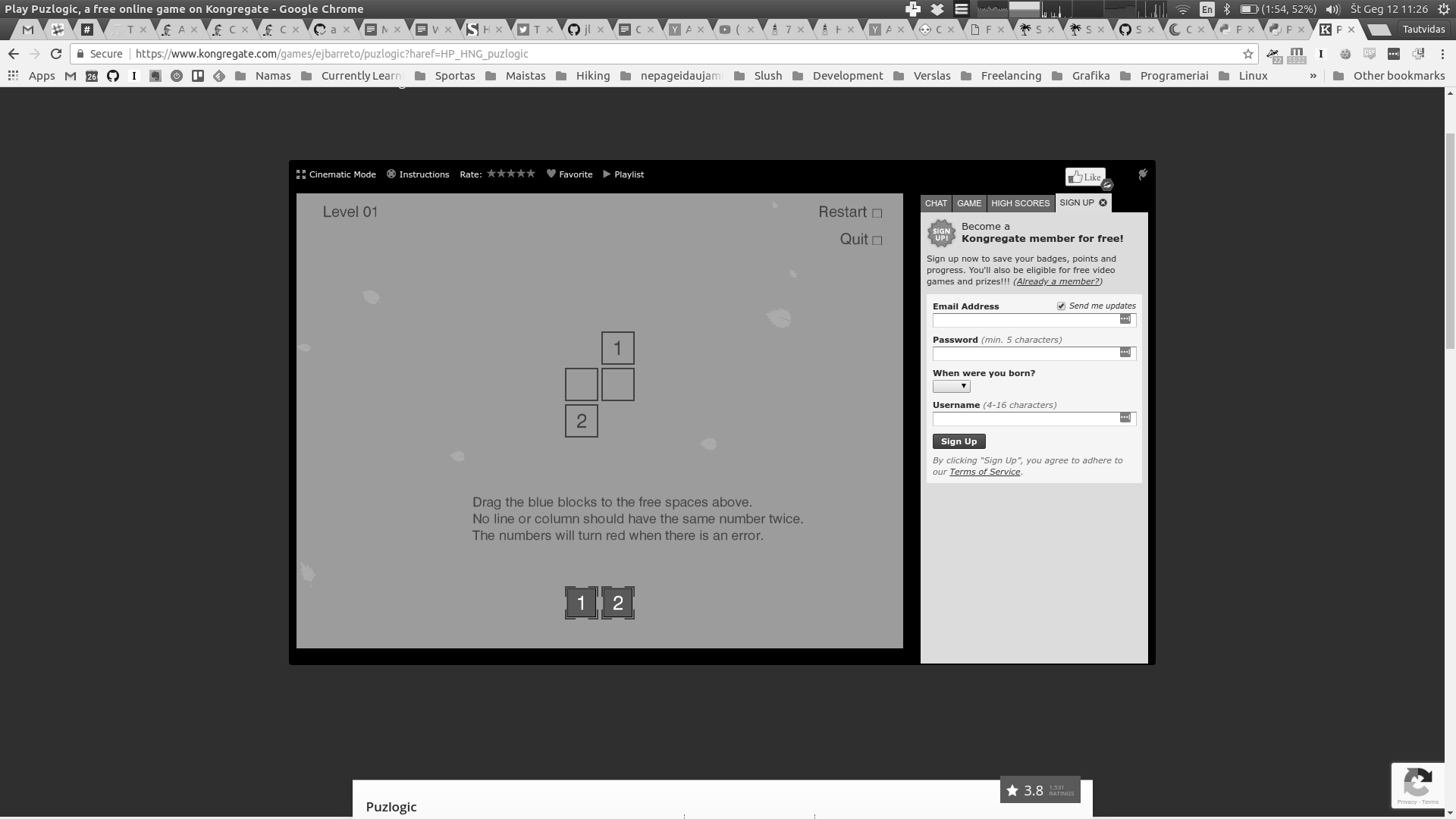3. Further simplify the image to binary grayscale, doing so eliminates most of the tiny details.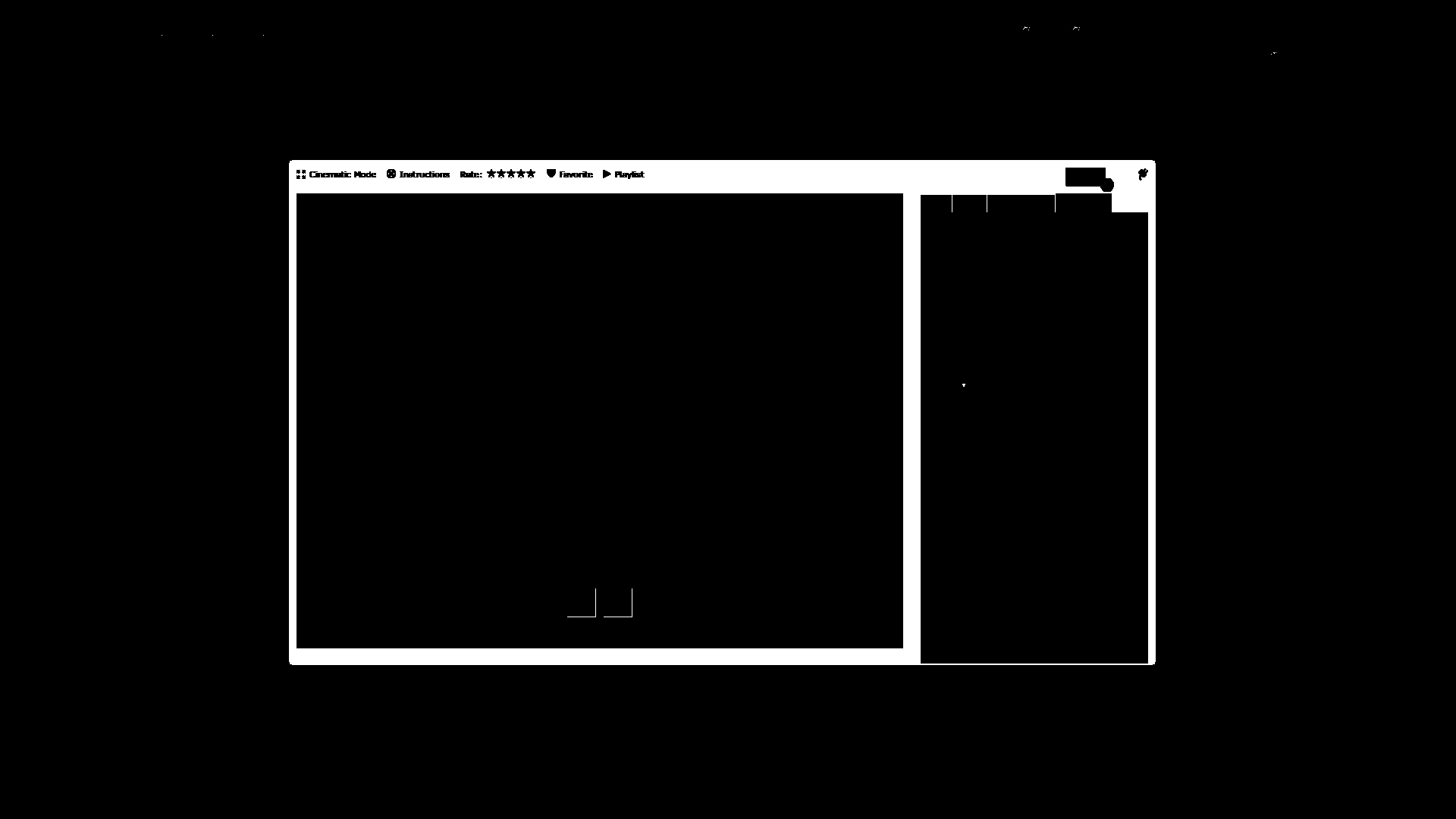4. Dilation further decreases the size of small details. Now we can start looking for contours.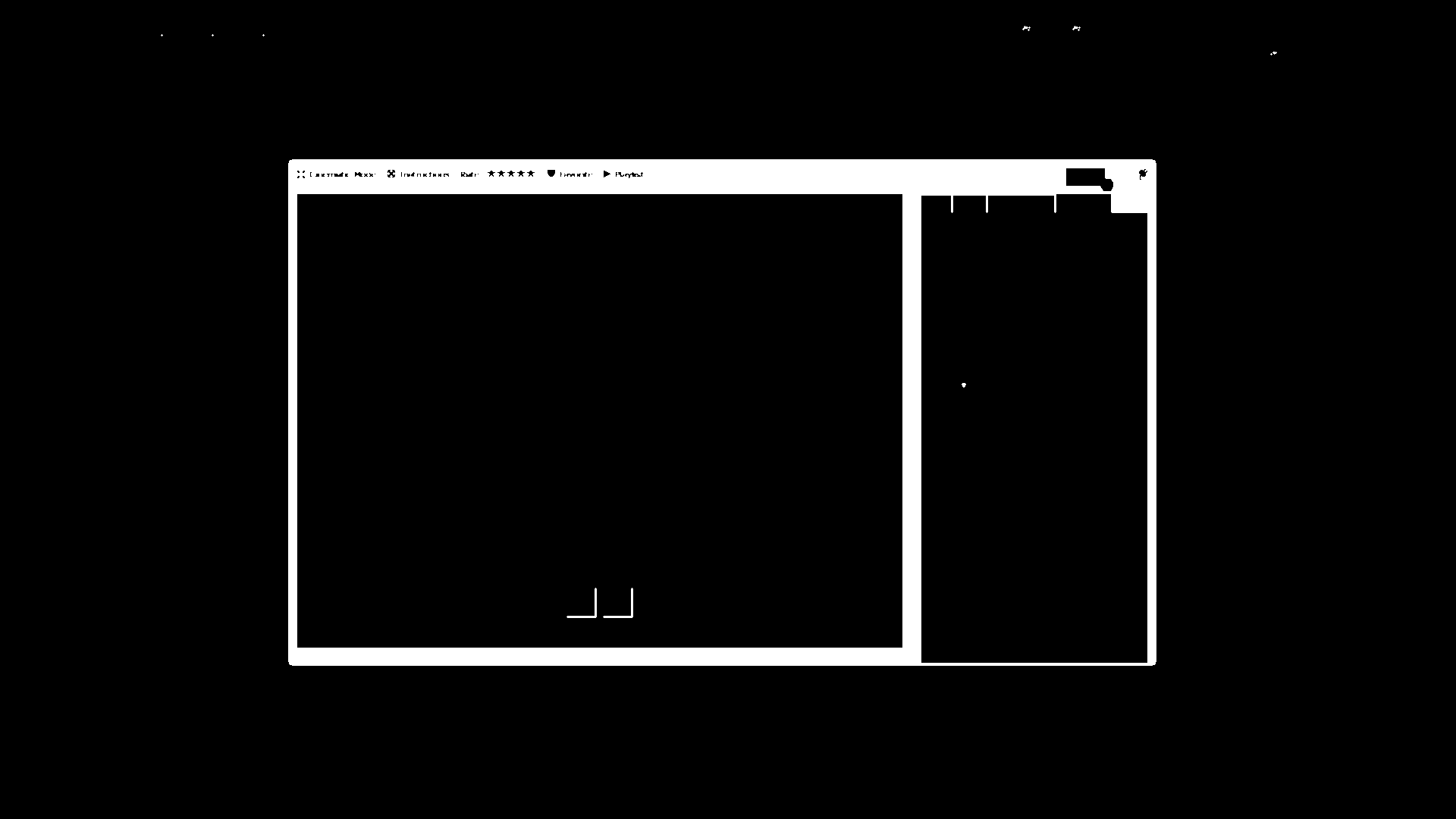5. As you can see, it finds quite a few contours, that’s why I tried to simplify the image as much as possible.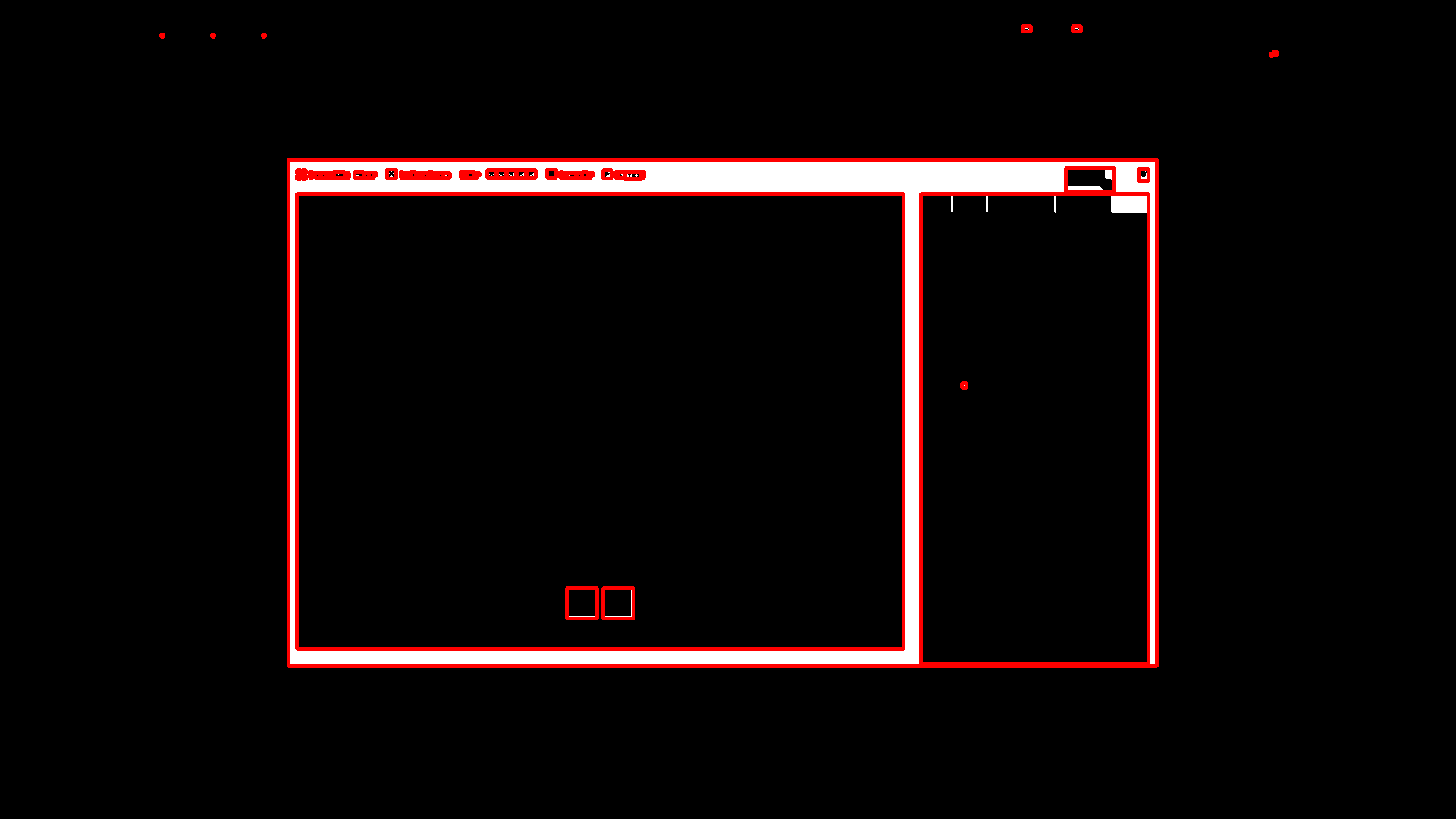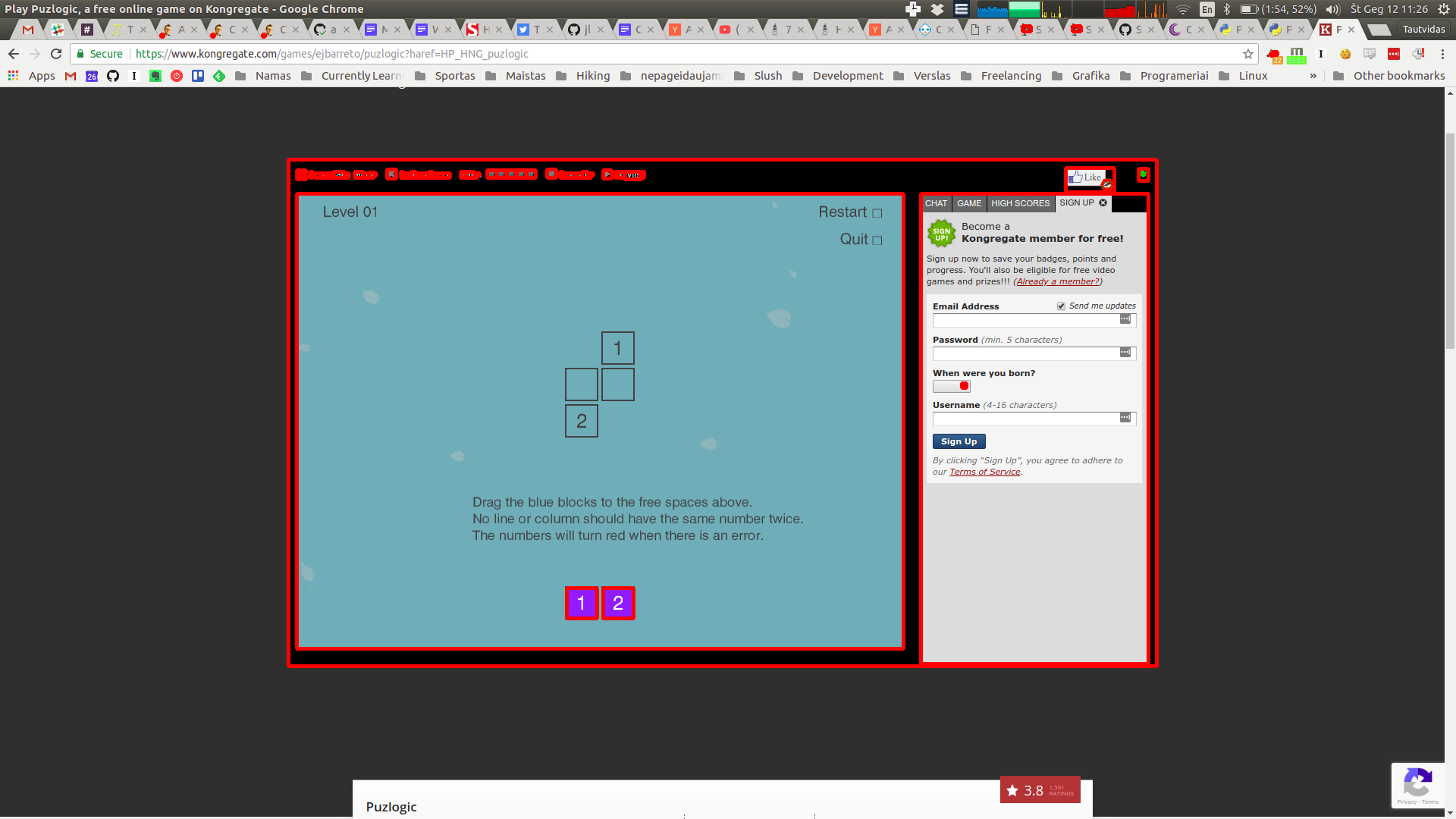6. Further filtering by bounding box’s dimensions allows to identify the game screen, as pictured above.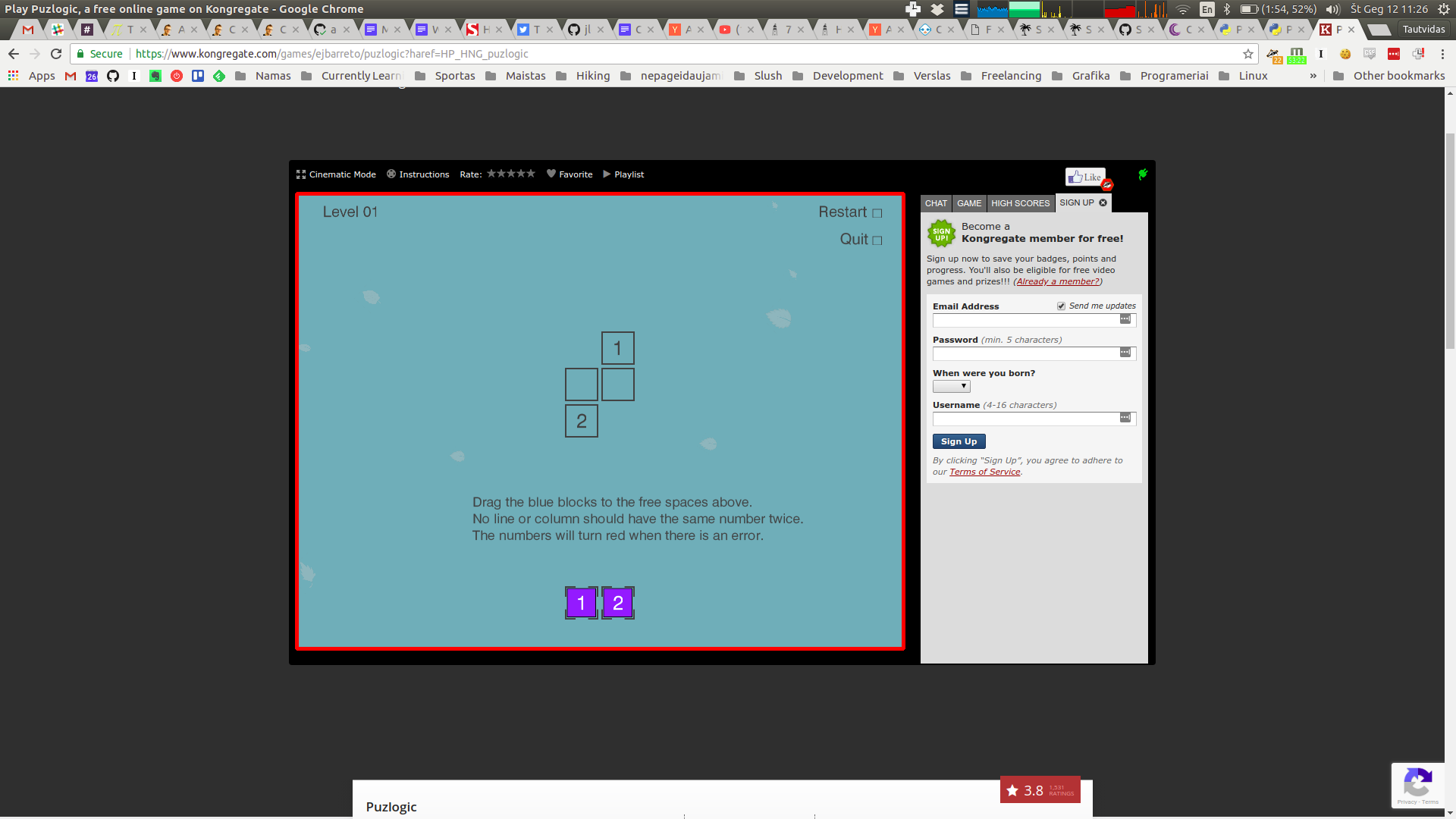7. And here it is cropped to a useable image for further processing: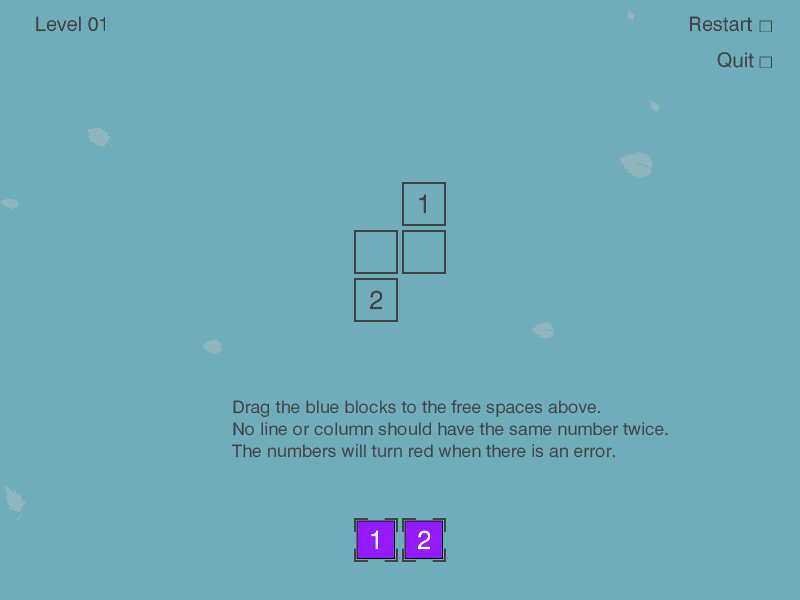The final result for this image is: `Board(x=391, y=255, w=800, h=600, screen=np.array(..., dtype=uint8))`

Lastly, for future processing we take only the area that actually is the game screen. We can ignore the rest of the screen for vision purposes, but we still have to keep in mind offsets of the game area to allow us control mouse movements later.

#### Finding all cells

Now that we have the game area as a cropped image, we can start looking for game cells within it. Both the game board (are where game pieces should be placed), as well as the pieces themselves are squares of a similar size, just colored slightly differently. So we can write the same method to look for all cells, and then separate target cells from game pieces.

The pattern is similar:

• Convert image to binary grayscale
• Dilate to eliminate some of the noise
• Find all contours, filter them to ones roughly matching the target size
``````def get_visible_cells(self):
board = self.get_game_board()

grayscale = cv2.cvtColor(board.screen, cv2.COLOR_BGR2GRAY)

ret, mask = cv2.threshold(grayscale, 100, 255, cv2.THRESH_BINARY)

# Erase smaller artifacts on screen, hopefully leaving only
# larger contours to detect.
kernel = cv2.getStructuringElement(cv2.MORPH_CROSS, (3, 3))
dilated = cv2.dilate(binary_grayscale, kernel, iterations=1)

_, contours, _ = cv2.findContours(dilated, cv2.RETR_EXTERNAL, cv2.CHAIN_APPROX_SIMPLE)

Cell = namedtuple('Cell', ['x', 'y', 'w', 'h', 'content'])

# [x, y, w, h, img]
bounding_boxes = map(lambda c: list(cv2.boundingRect(c)), contours)
candidates = filter(lambda b: 40 < b < 60 and 40 < b < 60, bounding_boxes)
cells = map(lambda c: Cell(c, c, c, c, self._recognize_number(board.screen[c:c+c, c:c+c])), candidates)
return list(cells)
``````

I’ve set contour sizes for cells to be bigger than 40px, but smaller than 60px. It was done mostly by trial and error. I think the actual size of the game piece is around 48px, but the game cell is slightly bigger, and we’ve done some dilation as well.

To calculate `cells`, we crop the source image based on coordinates of the countour’s bounding box, taking box’s width and height into account. Note that here I’m also using `self._recognize_number(...)` call, which does OCR of cell’s contents, turning it into an actual number, or returning `False`. We’ll get to it in a minute.

1. It all starts with the full game screen.2. This is how it looks like after turning the image into a binary grayscale image with some dilation. You can see that most of the details have been almost eliminated.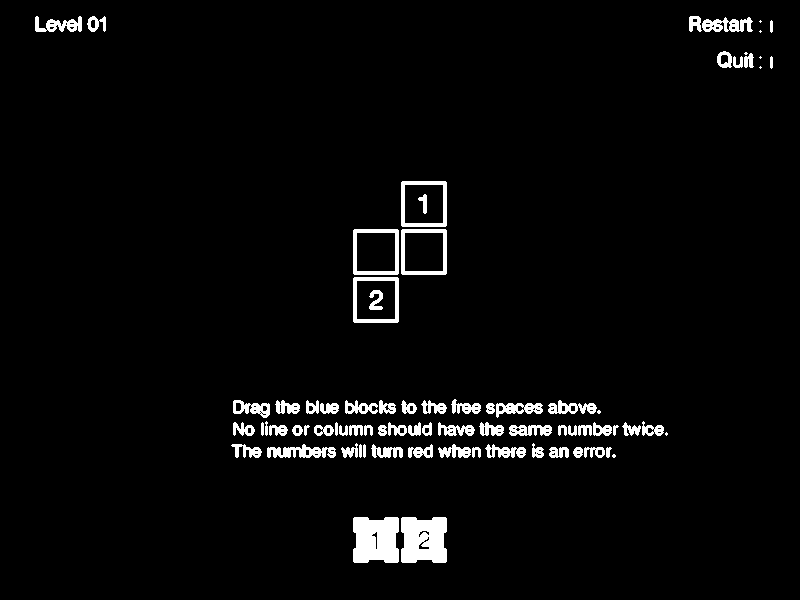3. We find all contours and filter them based on expected contour dimensions. After some trial and error that leaves only the cells.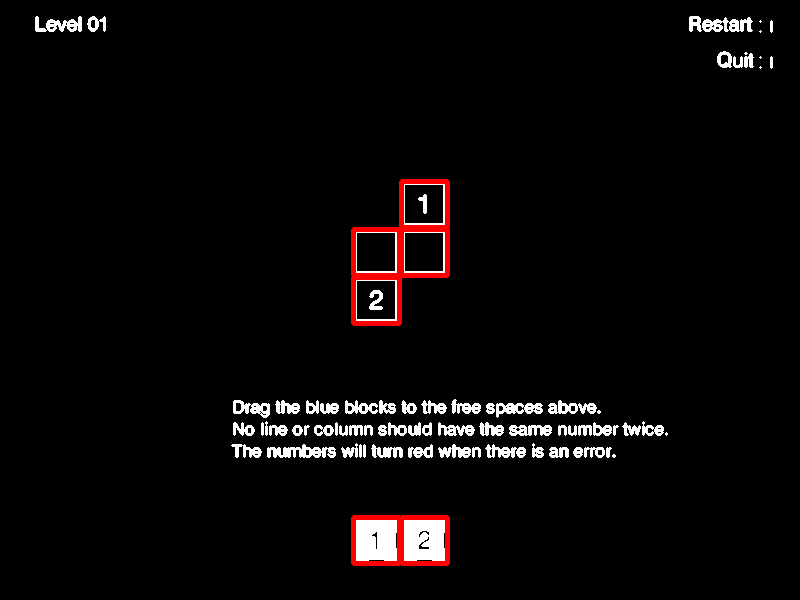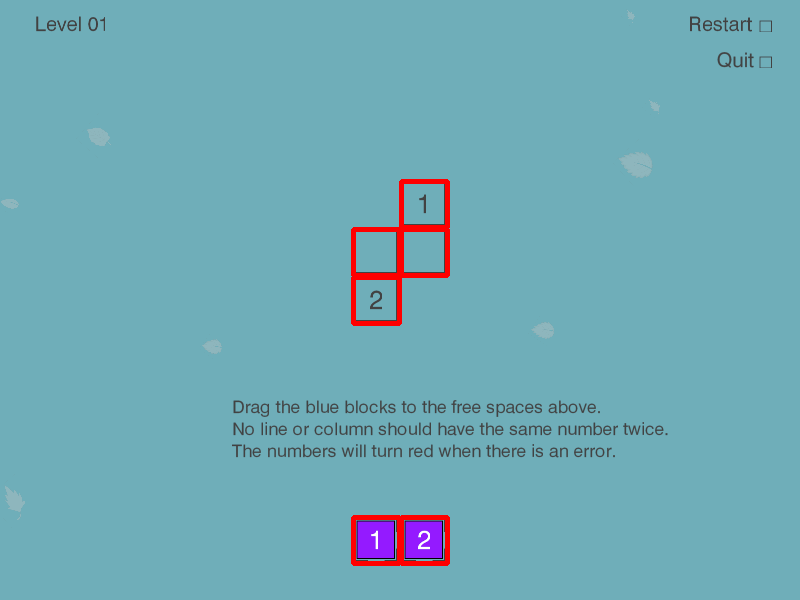Method’s result for this picture is:

``````[
Cell(x=401, y=517, w=46, h=46, content=2),
Cell(x=353, y=517, w=46, h=46, content=1),
Cell(x=353, y=277, w=46, h=46, content=2),
Cell(x=401, y=229, w=46, h=46, content=False),
Cell(x=353, y=229, w=46, h=46, content=False),
Cell(x=401, y=181, w=46, h=46, content=1)
]
``````

You can see that it was able to find all game board cells as well as all game pieces.

#### Finding game pieces

Now that we have all the cells identified, we can use this information to figure out which ones are game cells.

On different maps there may be different number of pieces provided in different arrangements, e.g. two rows with different number of pieces, two rows with same number of pieces, one row of pieces.

The common theme I saw in the first few levels was there were at most 2 rows of pieces, so I’ll assume the same will hold for all levels in the game. The other nice thing is that the distance between the lowest game board cell and the highest piece is fairly big, which we can use to differentiate cells from pieces.

Based on that information, we can find the lowest cell in the set of cells and assume that it is actually a game piece. Furthermore, I’ll assume that anything vertically within 3 cell heights of the lowest cell is also a piece.

``````def get_pieces(self):
cells = self.get_visible_cells()
lowest_cell = max(cells, key=lambda c: c.y)

# Expect available pieces to be lower than the lowest row of game board cells
return list(filter(lambda c: abs(lowest_cell.y - c.y) < lowest_cell.h*3, cells))
``````

With the original image this method will return:

``````[
Cell(x=401, y=517, w=46, h=46, content=2),
Cell(x=353, y=517, w=46, h=46, content=1)
]
``````

#### Finding game board cells

Now that we have identified which cells are actually movable pieces, any other cell must then be a game board cell!

``````def get_cells(self):
cells = self.get_visible_cells()
pieces = self.get_pieces()

return list(set(cells) - set(pieces))
``````

Result of this method would be:

``````[
Cell(x=401, y=181, w=46, h=46, content=1),
Cell(x=353, y=229, w=46, h=46, content=False),
Cell(x=353, y=277, w=46, h=46, content=2),
Cell(x=401, y=229, w=46, h=46, content=False)
]
``````

#### Recognizing digits within cells

Above we identified some cells, which may be game board cells, or game pieces. So the cell may contain a number, or it may not.

The procedure is similar to what we’ve done before, but there are some differences.

For OCR we will use Pytesseract bindings for Google’s Tesseract. Tesseract works best when we feed in images containing just black text on white background with as little noise and unnecessary elements as possible.

Due to that the first step is to remove the black border around the cell that some cells may have, as I found it would be easier for OCR to reliably recognize the digit.

Next the image needs to be converted to binary grayscale, as the cell may contain other colors (like purple background of the piece, colored background of the cells). At this point we should have either black text on white background, or white text on black background.

Previous steps may have made edges of any digits a little pixelated, so blurring the image should make the digit more solid and slightly bigger, giving a better chance for OCR to recognize it.

Last step before feeding the image to OCR, we need to make sure the image contains black text on white background. I tried feeding in white text on black background, but it didn’t work well, and at this point the image may contain black text on white background or vice versa.

To make sure the image is actually white text on black background, we check the color of the first pixel. If it is white (has a value of `255`) - I assume that it’s black text on white background. But if the pixel is black (has a value of `0`), then I assume it’s white text on black background, so we have to invert image’s colors.

Lastly we feed in the image to pytesseract and attempt to convert the result into an integer. The `--psm 10` argument for Tesseract instructs that the image should contain just a single character inside. In other modes I found that Tesseract returned mostly unusable garbage.

``````def _recognize_number(self, candidate_tile_image):
""" Attempts to OCR the number within a game tile image """

borderless_image = candidate_tile_image[5:-5, 5:-5]
grayscale = cv2.cvtColor(borderless_image, cv2.COLOR_BGR2GRAY)

ret, mask = cv2.threshold(grayscale, 100, 255, cv2.THRESH_BINARY | cv2.THRESH_OTSU)

# Blur to help text show up better for OCR
ocr_image = cv2.medianBlur(binary_grayscale, 3)

if ocr_image[0, 0] == 0:
# OCR needs black text on white background
black_text_on_white_background = cv2.bitwise_not(ocr_image)
ocr_image = black_text_on_white_background

# Use single-character segmentation mode for Tesseract
character = pytesseract.image_to_string(ocr_image, config='--psm 10')
try:
return int(character)
except:
return False
``````
1. We start with the image of an isolated cell of the original game screen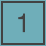2. This is how the cell looks like after turning it into a binary grayscale image and removing its borders. Note that background disappears as well.3. Here’s how the image looks like after slight blurring. The digit looks slightly bolder, but it seems for this image blurring may not have been all that necessary.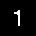4. Because the image was white text on black background, the last step was to invert the colors and feed the resulting black-on-white image into Tesseract for OCR.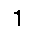And here is the final result of the method is: `1`. A parsed integer digit.

#### Performance tuning

Now that we have most of the basic vision done, we can run some tests on the actual game, and it does work. However, when performing such commands in rapid succession, it may take in the region of 10 seconds on my machine for all calls to respond.

Mostly because some calls use others, so there’s quite a bit of unnecessary re-processing happening. To improve the situation we’ll add basic caching: cache results of each class method for the given frame, clear out the cache on the next frame.

For caching I’ve used decorators. Set a `cache` property dict on the object, adding return values to the cache when computation happens.

``````def cache_until_refresh(func):
def wrapper(self):
if func in self.cache:
return self.cache[func]

result = func(self)
self.cache[func] = result
return result

return wrapper

class Vision:
def __init__(self, source):
self.source = source
self.cache = {}

def refresh(self):
self.cache = {}
self.source.refresh()

@cache_until_refresh
def get_game_board(self):
# ...

@cache_until_refresh
def get_pieces(self):
# ...

@cache_until_refresh
def get_cells(self):
# ...

@cache_until_refresh
def get_visible_cells(self):
# ...
``````

Now instead of having to wait for full results 10 seconds, the response comes in under 2.

### Closing thoughts

So now we have a basic way to identify the game area, know which pieces are available, where are the target cells for game pieces, as well as which number each cell has.

Of course, the methods used above are quite brittle, at least as implemented here. Changes in UI, scaling, adding more complex or just other elements may easily break the bot. But, you can also see how powerful these simple-once-abstracted techniques are: convert to grayscale, dilate/blur, find contours/bounding boxes and one can identify objects without providing a reference picture of it. A more sophisticated approach than image pattern matching in Burrito Bison bot I wrote about previously.

If you are interested in the full code mentioned in this post, you can find it on Github - vision.py. The full project’s code, possibly in a further state of the project, can be found on Github - flakas/puzlogic-bot

In the next post we’ll look into adding Mouse controls and tying it all together to get the bot to actually solve several puzzles. A demo video shall follow too.

• ## Solving Puzlogic puzzle game with Python and OpenCV: Part 1

In the previous post we looked into applying basic pattern matching with OpenCV to build a basic bot to play Burrito Bison browser game. However, there was very little game logic involved: it watched and waited for powerups to fill up, using them once filled up. Everything else was related to keeping the game flow going by dealing with menus.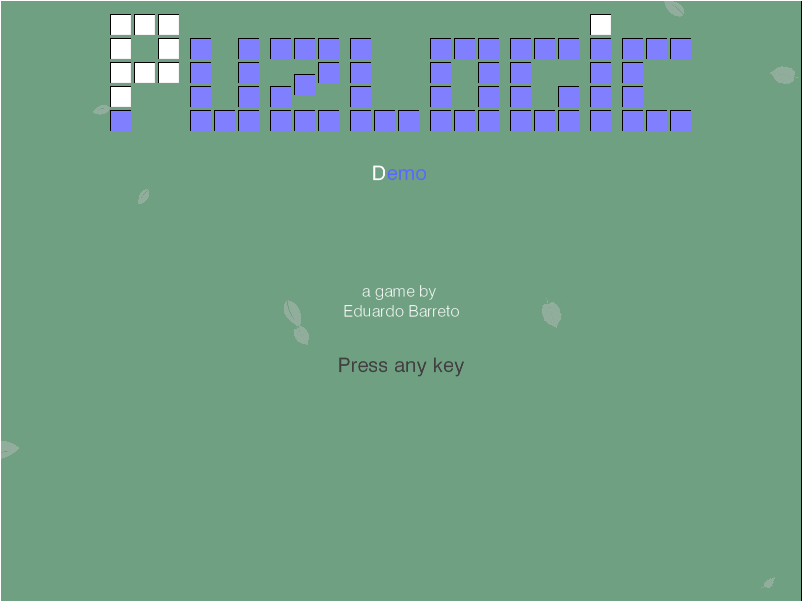In this post (or next few posts) let’s take a look at a slightly different game: Puzlogic. Puzlogic is somewhat similar to sudoku and comes with 15 different levels. The goal of the game is to place a set of given numbers into a grid where some cells are pre-filled with other numbers. The catch is that duplicates are not allowed in any column or row, and in some cases extra constraints can be applied, e.g. that numbers on a given column or row would add up to a specific sum.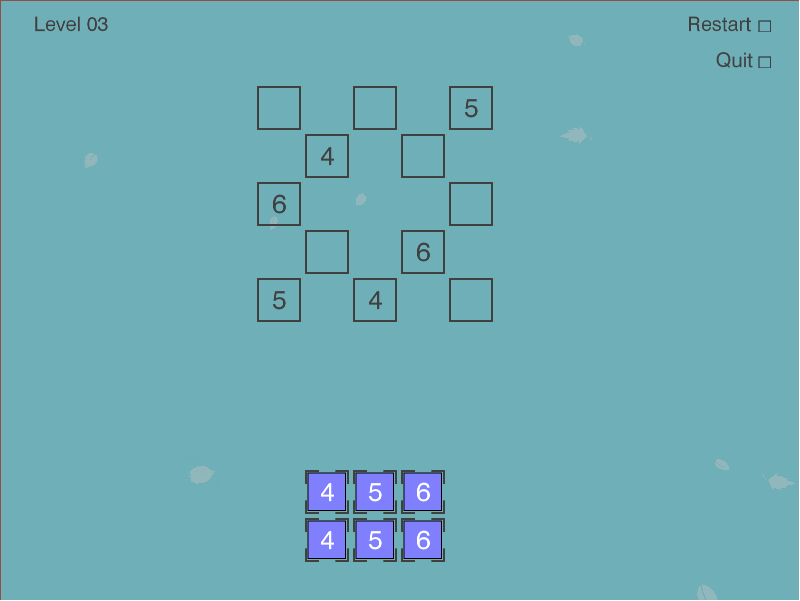I would recommend you to play the game for several rounds to familiarize with it.

But I think Puzlogic is a convenient problem to explore several approaches on:

1. More elaborate computer vision approaches to understand the grid, e.g. recognition of digits, identifying different elements (irregularly spaced grid, set of given numbers, sum constraints)
2. Solve the puzzle using Python, e.g. using a bruteforce approach, or using a theorem prover like Z3.
3. Lastly, combine the two components above and use the a mouse controller like Pynput to tie it all together.

In this first post let’s start with the puzzle solver first, tackling CV and controls portion in later posts.

### Planning

Again, at first I’d recommend completing a first few puzzles manually to learn how the game works. But here are my first impressions: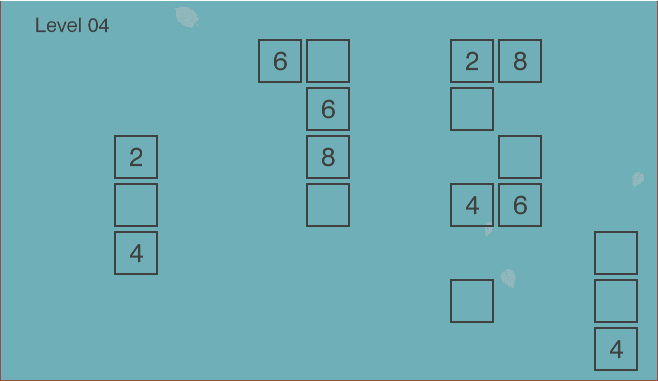The game board is in the form of the grid. However, not every location in the grid is a valid cell - there may be spaces around valid cells. Some valid cells have pre-filled numbers in them which cannot be overwritten, while empty cells are intended for the player-controlled pieces.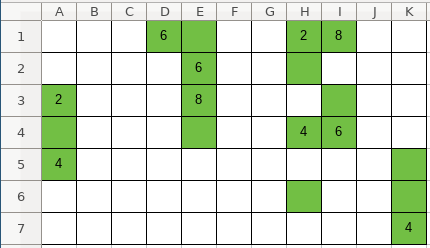Since it is a grid, we can think about it as an `NxM` matrix, where `N` and `M` are distances between furthest horizontal and vertical valid cells. However, because most cells may not be valid, it would be a waste of space to represent it as a two-dimensional array. Instead it can be represented as a Sparse matrix using a coordinate list, where each cell is represented as `(x_coordinate, y_coordinate, value)` triplet. So the full grid would be represented as: `[(x_0, y_0, value_0), (x_1, y_1, value_1), ... (x_n, y_n, value_n)]`.

Secondly, the numbers player has to use (we’ll call them “pieces”) are displayed near the bottom of the window in another grid, which means coordinates will be important later when moving the pieces to the game board. That can be represented as a simple list of integers: `[piece_0, piece_1, ..., piece_n]`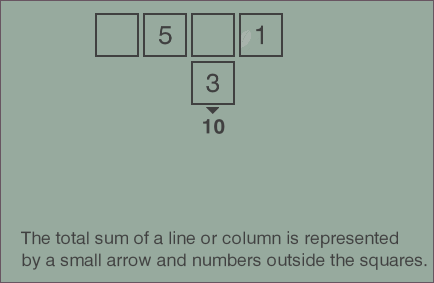Third, if you play a few more rounds of the game, you’ll see how sum constraints are displayed. They can be applied to any row or any column. We can represent the constraint with another triplet: `(index, 'column', target_sum)` or `(index, 'row', target_sum)`. The list of constraints then would be represented as: `[(index_0, dimension_0, target_0), ..., (index_n, dimension_n, target_n)]`.

So we have our three parameters: grid, pieces and constraints.

And lastly, how would a solution be represented? The game is won once all the game pieces are moved to the game board, the game board is valid and all constraints are satisfied. So we could represent the solution as a list of moves, where a move is a triplet `(x, y, piece)`, denoting on which coordinates each piece should be placed.

The number of pieces is low, the size of the game board is also small, so we can use a brute force depth-first search to find at least one solution.

### Implementation

For pseudocode of the algorithm, I think a pattern I’ve seen used during Martin Odersky’s Scala classes works well here:

1. Generate all possible moves for the given position;
2. Filter the list of all moves leaving only the legal moves;
3. Recursively repeat from step 1 with the new game state.
4. If the solution is found - recursively return it through the execution tree, if not - allow the depth-first search to continue until the whole search space is exhausted.

Let’s start at the top with `solution()` and fill in the blanks:

``````    def solve(self, board, pieces, constraints=[]):
if len(pieces) == 0:
return []

for (move, new_board, new_pieces, new_constraints) in self.legal_moves(board, pieces, constraints):
solution = self.solve(new_board, new_pieces, new_constraints)
if solution != False:
return [move] + solution

return False
``````

This is following the exact same pattern as outlined above: generates new states for each of the legal moves, and continues exploring the new legal states one by one. If the solution has been found - the current move would be added to the solution. If not - the search continues until exhaustion.

`legal_moves` is missing, so let’s implement it next. What it has to do is for each piece in the `pieces` list generate a new state (board, remaining pieces) as if we made the move. Constraints don’t really change, so we can return the same argument. As per above, it would need to return the quadruplet: `(move, new_board, new_pieces, constraints)`.

``````    def legal_moves(self, board, pieces, constraints):
return (move for move in self.all_moves(board, pieces, constraints) if self.is_legal(move, constraints))
``````

Here a generator expression is used to avoid having to store all possible moves in memory at once as search space is explored at each recursion depth. Instead the first matching value will be generated, the generator will return, search will continue in the deeper search level.

Subsequently, `all_moves()` can be defined as:

``````    def all_moves(self, board, pieces, constraints):
""" Attempt to put one of available pieces into the available spaces on the board """
free_cells = [(c, c) for c in board if c == 0]
return (
((row, column, piece), new_board, new_pieces, constraints)
for (row, column) in free_cells
for piece in pieces
for (new_board, new_pieces, new_constraints) in [self.perform_move((row, column, piece), board, pieces, constraints)]
)
``````

All it does is it attempts to place one of the available pieces into one of the free cells on the board.

When making a move, the selected piece is no longer available in the `pieces` list for further use. Instead it’s placed into the `board` matrix at given coordinates, making it permanent for deeper recursion to work with.

``````    def perform_move(self, move, board, pieces, constraints):
""" Moves the given piece to the location on the board """
new_pieces = pieces.copy()
new_pieces.remove(move)

new_board = board.copy()
new_board.remove((move, move, 0))
new_board.append(move)

return new_board, new_pieces, constraints
``````

Next let’s take care of `is_legal()`, which evaluates whether a given board is legal:

``````    def is_legal(self, board, constraints):
"""
Is the board legal.
- Rows and columns contain no duplicates
- If there are constraints and all cells are filled in a given column - the sum of the column does not exceed the constraint
- If all cells are filled in - constraint matches
"""

lines = self.rows(board) + self.columns(board)

no_duplicates = all(self.all_unique(self.filled_cells(line)) for line in lines)
satisfies_constraints = all(self.satisfies_constraint(board, c) for c in constraints)

return no_duplicates and satisfies_constraints
``````

The uniqueness constraint in rows and columns:

``````    def all_unique(self, line):
return len(line) == len(set(line))

def rows(self, board):
return self._lines(board, 0)

def columns(self, board):
return self._lines(board, 1)

def _lines(self, board, dimension=0):
discriminator = lambda c: c[dimension]
cells = sorted(board, key=discriminator)
groups = itertools.groupby(cells, key=discriminator)
return [[c for c in group] for index, group in groups]

def filled_cells(self, line):
return [x for x in line if x != 0]
``````

The end goal of the summing constraint is to make sure that once all cells are filled in a line that the target sum is reached. However, in order for us to perform the search there will be times when some cells are not filled in. In such cases we’ll consider the constraint satisfied only if the sum of a line is lower than the target sum - there’s no point in continuing the search if we already blew over the limit.

``````    def satisfies_constraint(self, board, constraint):
(dimension, element, target_sum) = constraint
line = self._lines(board, dimension)[element]
line_sum = sum(line)
return (
(line_sum == target_sum and self.all_cells_filled(line))
or
(line_sum < target_sum)
)
``````

### That’s it!

And that’s it! We should have a working solver for the puzzle. Of course, having tests would be good to make sure it actually works and keeps on working, and I’ve already done that in the project’s repo.

Now we can test it out with the first level:

``````solver = Solver()
board = [
(0, 1, 1),
(1, 0, 0),
(1, 1, 0),
(2, 0, 2)
]
pieces = [1, 2]

moves = list(solver.solve(board, pieces, []))
print(moves)

# > [(1, 0, 1), (1, 1, 2)]
``````

Or the third level:

``````solver = Solver()
board = [
(0, 0, 0),
(0, 2, 0),
(0, 4, 5),
(1, 1, 4),
(1, 3, 0),
(2, 0, 6),
(2, 4, 0),
(3, 1, 0),
(3, 3, 6),
(4, 0, 5),
(4, 2, 4),
(4, 4, 0),
]
pieces = [4, 5, 6, 4, 5, 6]

moves = list(solver.solve(board, pieces, []))
print(moves)

# > [(0, 0, 4), (0, 2, 6), (1, 3, 5), (2, 4, 4), (3, 1, 5), (4, 4, 6)]
``````

Looks like it works! While this is not a very elegant approach, a brute force approach with small numbers still seems sufficient, which is great for the toy application. In the next post I’ll start looking into the computer vision part.

If you’d like to see the full code of the solver, you can find it on the project’s Github repo: puzbot - solver.py.

• ## Certbot renewal of Let's Encrypt certificate fails with "Failed authorization procedure" on CloudFlare

Let’s Encrypt has taken certificate business by storm, issuing over 50 million active certificates (stats). It is free and easy way to start using HTTPS on your website to secure the traffic.

One slight inconvenience is that certificates themselves are fairly short lived (only 90 days) and require renewals. Luckily, there are automated tools like Certbot which help you handle renewals. If you have it set up just right, you can create a cron job and it will continuously renew the cert for you. E.g. I use geerlingguy.certbot Ansible role for it.

However, recently I noticed that the renewal started failing with the following error message:

``````Attempting to renew cert (hermes.tautvidas.lt) from /etc/letsencrypt/renewal/hermes.tautvidas.lt.conf produced an unexpected error: Failed authorization procedure. hermes.tautvidas.lt (tls-sni-01):
+urn:acme:error:tls :: The server experienced a TLS error during domain verification :: remote error: tls: handshake failure. Skipping.
All renewal attempts failed. The following certs could not be renewed:
/etc/letsencrypt/live/hermes.tautvidas.lt/fullchain.pem (failure)
1 renew failure(s), 0 parse failure(s)
``````

As per this ticket, Let’s Encrypt will try to match the requester server’s IP address with given domain’s DNS A record IP:

``````To fix these errors, please make sure that your domain name was
entered correctly and the DNS A/AAAA record(s) for that domain
firewalls are preventing the server from communicating with the
client. If you’re using the webroot plugin, you should also verify
that you are serving files from the webroot path you provided.
``````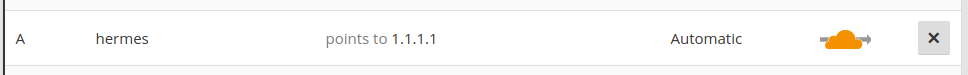However, if you have CloudFlare enabled for that domain and you have it configured to run in HTTP Proxy mode - CloudFlare might be interfering. That is because of how CloudFlare CDN works: visitors will connect to CloudFlare’s servers, and if CF can - it will return them the requested resource without ever passing the request to your servers, if it cannot - it might request your server for that resource. But whatever happens, the visitor will see that it is connecting to CloudFlare’s servers - not yours!

So if you do have CloudFlare’s HTTP Proxy enabled, consider disabling the Proxy mode for that particular (sub)domain: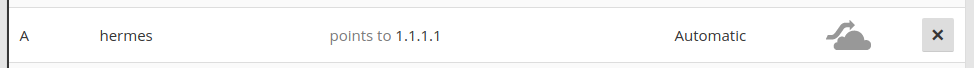You should be able to renew the certificate after doing so.

However, if you cannot disable CloudFlare’s HTTP proxy for your domain, consider using CloudFlare’s Origin Certificate to encrypt communication between your server and CloudFlare, and use CloudFlare’s Universal SSL between CloudFlare and your visitors.

• ## Google Code Jam 2018: Qualification round results

Last time I participated in the Practice round of Google Code Jam 2018. It was a fun experience, so on 2018-04-07 I went for the Qualification round.

While practice round took about 7 hours to complete, the qualification round topped that requiring about 10h of work. As previously, there 4 problems with one visible and one hidden result each. I managed to correctly complete 1/2 tests for the first three, and then 2/2 tests for the fourth one for a total of 55/100 points. Bummer!

But, here’s how I approached the problems.

### 1. Saving the universe again

In short, a killer robot is executing a program, which consists of 2 instructions. `C` charges the weapon, doubling the damage, `S` shoots the weapon. Hero can swap two adjacent instructions, but want to do so as few times as possible. What is the minimum number of such changes have to be made so that the robot doesn’t do more than a specific amount of damage? E.g. `SCSS`: shoot for 1 damage, charge damage to two, shoot for 2 damage (`total=1+2=3`), shoot for 2 damage (`total=1+2+2=5`).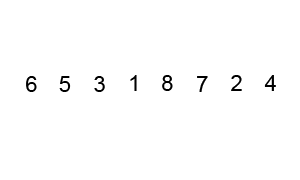By Swfung8 - Own work, CC BY-SA 3.0

To start with, having to swap instructions around sounds similar to Bubble Sort, where you swap around adjacent elements if left one is bigger than the right one. Here idea is similar as well.

The second point we can notice is that `CHARGE` instruction doubles damage for any future `SHOOT` instructions, but does not do any damage by itself. So a) any `CHARGE` instruction after the last `SHOOT` instruction is irrelevant and can be discarded from the search, and b) we can move `CHARGE` instructions to the right.

There is a special case to account for: when is it impossible to stay under a specific amount of damage? And the limit is that if all `SHOOT` instructions are on the left hand side (and are worth 1 point each), if that exceeds the damage limit - there’s nothing else we can do to avoid that damage. So the first step is to check if it’s even possible to achieve the intended damage target:

``````def count_instructions(instruction, instructions):
return instructions.count(instruction)

def solve(max_damage, instructions):
if count_instructions(SHOOT, instructions) > max_damage:
return 'IMPOSSIBLE'
# ...
``````

As for the core behavior, which instructions would affect the end result the most? Well, `CHARGE` instructions stack, so if you had 5 `CHARGE` instructions and then a `SHOOT` instruction - that’s `2 ^ 5 = 32` damage points. But, if we swap the last `CHARGE` instruction with `SHOOT` instruction - that cuts damage in half (`2 ^ 4 = 16`).

So the main algorithm is as follows:

1. Trim any trailing `CHARGE` instruction, that don’t have a `SHOOT` instruction after them. We’ve already established it - they don’t affect the end result;
2. Find the rightmost `CHARGE` instruction that has a `SHOOT` instruction following it, and swap the two. Doing so will reduce the power of that `SHOOT` instruction by 50% - the most we can achieve at that point;
3. Repeat until the estimated damage is low enough.
``````def prune_instructions(instructions):
last_instruction = instructions[-1]
while instructions != [] and last_instruction != SHOOT:
instructions.pop()
last_instruction = instructions[-1]
return instructions
``````
``````def swap_with_left(index, instructions):
start_len = len(instructions)
instructions[index-1:index+1] = [instructions[index], instructions[index-1]]
return instructions

def swap_last_shoot_with_charge(instructions):
instructions.reverse()
first_charge = instructions.index(CHARGE)
instructions = swap_with_left(first_charge, instructions)
instructions.reverse()
return instructions
``````
``````def solve(max_damage, instructions):
if count_instructions(SHOOT, instructions) > max_damage:
return 'IMPOSSIBLE'
max_changes = 100
changes = 0

while count_damage(instructions) > max_damage and changes < max_changes:
instructions = prune_instructions(instructions)
instructions = swap_last_shoot_with_charge(instructions)
changes += 1

return changes
``````

And that’s it. However, this passed only 1 out of 2 tests.

Looking at it again, it may be due to me limiting `max_changes` to `100` - I forgot to consider that number of instructions can be as high as `30`. Meaning that if four rightmost instructions are `SHOOT` - the algorithm would prematurely terminate, yielding a wrong answer. Gah! It should’ve been a bit higher, maybe `10e3` or `10e4`.

### 2. Trouble sort

A sorting algorithm is given in pseudocode, and it’s not functioning correctly. Our job is to write a program that would check its results, writing `OK` if it worked normally, or outputting the index of an element preceeding a smaller number.

Sounds simple enough. We could memorize the first element of the sorted list, loop through the sorted list, making sure that the current element is not smaller than the previous one. If there is a failure - we found the problem with the previous element. If it passed - we return `OK`.

``````# Given in problem description
def trouble_sort(L):
done = False
while not done:
done = True
for i in range(len(L)-2):
if L[i] > L[i+2]:
done = False
temp = L[i:i+2+1]
temp.reverse()
L[i:i+2+1] = temp

return L

def solve(N, values):
L = trouble_sort(values)

last_value = L
for (i, x) in list(enumerate(L))[1:]:
if last_value > x:
return i-1
last_value = x

return 'OK'
``````

This passed the first visible test, but failed the second hidden test, exceeding the time limit. What I forgot to take into account is the “special note”:

Notice that test set 2 for this problem has a large amount of input (`10^5` elements), so using a non-buffered reader might lead to slower input reading. In addition, keep in mind that certain languages have a small input buffer size by default.

So the solution exceeded the 20 second time limit. Not sure yet if input is really the full problem, e.g. generating the full enumerated list is not ideal, it would’ve been smarter to leave the generator as is, computing values lazily. But still, that’s another blunder.

### 3. Go, Gopher!

In short, we are given a number of cells that need to be marked. But, the way these cells have to be oriented in a grid has to form a continuous rectangle with no gaps. E.g. for input of `A=20` one might choose a grid of `4x5` rectangle. The tricky part is how cells can be marked. The program has to specify which cell it wants to mark, but the testing program can mark that or any one of its 8 neighboring cells randomly, telling you which cell has been actually marked. And even if a cell has already been marked - the Gopher may mark it multiple times. Doing so doesn’t do anything extra, only wastes the guess.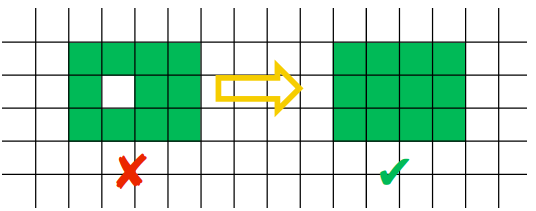I don’t know how to deal with randomness in such a case. Perhaps one could try gaming the pseudorandom number generator, but it’s unlikely.

A perhaps more likely approach could be to start with a couple arbitrary guesses, compute probabilities for available cells find the next best guess that way, and try to evolve the grid to fit into desirable shape with a specific area. But such approach sounds complex, so instead I went for the (very) naive approach.

The RNG guesses uniformly, so probability that each of the 3x3 cells gets prepared is `1/9`. So we could orient the `3x3` block in such a way that it always fits within the area we want to fully mark, and move the block from side to side, up and down, keeping guessing until we’re lucky and we fill the intended grid, or we run out of guesses. On the first visible test where `N=20` it’s pretty likely that we’ll fill up a `4x5` grid, but I’m not so confident about the `N=200` and `14x15` grid.

Something to keep in mind is how much of an overlap there is between two adjacent block guesses. The larger the overlap - the more likely overlapped cells will get marked, but the fewer guesses there are to cover the rest of the board. And edges and especially corners are a problem for such approach, as e.g. for a corner to be filled in there will only be as many opportunities as times one can cover the full board.

I first tried moving one column and one row at a time, and that was enough to pass the interactive local test and the visible test. But I was worried about corners and edges of the rectangle for the `N=200` case, so instead I opted moving two columns and two rows at a time, reducing overlap between 2 adjacent guess blocks from 2 cells to 1 cell, but increasing the number of times the full board can be covered by about a factor of 2.

``````MAX_TRIES = 1000
HALT = 'HALT'

def has_test_failed(coords):
return coords == [-1, -1]

def has_test_succeeded(coords):
return coords == [0, 0]

def mark_filled_cell(cell, board):
board[tuple(cell)] = True
return board

def coords_offset(center, offset_x, offset_y):
return (center+offset_x, center+offset_y)

def get_cell(coords, board):
return tuple(coords) in board

""" List of cells in a guess block with a given center cell """
result = []
for i in range(3):
for j in range(3):
result.append(get_cell(coords_offset(center, i-1, j-1), board))
return result

def solve(A):
width = math.floor(math.sqrt(A))
height = math.ceil(math.sqrt(A))
WINDOW = 3
board = {}
guess = 0

while guess < MAX_TRIES:
# Leave out edges
w = list(range(width))[1:-1]
if width % 2 == 0: w.append(width - 1)
h = list(range(height))[1:-1]
if height % 2 == 0: h.append(height - 1)

for i in w:
for j in h:
center = (i+1, j+1)
# Given block is fully filled up
continue

if has_test_failed(filled_cell):
return HALT
if has_test_succeeded(filled_cell):
return
board = mark_filled_cell(center, board)
guess += 1
return HALT
``````

This passed 1 out of 2 tests, failing with a “Wrong answer” on the “N=200” hidden test. I didn’t expect the second one to pass - it was unlikely to do so. But, as a third option I would have tried placing guess blocks without any overlap at all, increasing the number of full rectangle guess coverage even further, in the hopes of hitting the right chance.

### 4. Cubic UFOIn short, there is a 3D cube hanging above ground and casting a shadow. How should we rotate the cube to get its shadow’s area to be as close as possible to a specific number? For simplicity’s sake, the cube’s center point is at origin (0, 0, 0), cube is 1km wide, and its shadow is being cast onto a screen 3km under the origin.

It was actually fairly straightforward, but tough to implement, requiring knowledge of multiple algorithms and implementation of matrix operations. I think I’ve spent most of my time on this problem.

The first idea was that maybe there is a mathematical formula describing an area of any cube slice through its center point. A quick search hasn’t yielded much, so this idea was abandoned.

Instead the requirements of the problem suggested a different approach. Notice that precision of answers has to be within about `10e-6`, and inputs for all test cases are very precise, e.g. (`1.000000`, `1.414213`, `1.732050`). This raised an idea from numerical methods class - maybe if we could rotate the cube slightly, we could discover directions in which rotation gave the closest shadow area, and following those directions would get closer and closer to the desired answer.

From numerical methods class I vaguely remembered Newton’s method and from neural networks class came Gradient Descent.

On the right path, allowing to get closer and closer to the solution, but it’s only part of the solution. Full implementation still requires knowing how to calculate verteces and faces of the rotated cube, project those points onto 2D space, and calculate the area of cube’s shadow.

#### 4.1. Cube rotations

First we need a way to describe the relevant coordinates. What would be the coordinates of each of the verteces and faces?

Well, cube’s center point is at origin, and the cube itself is 1km wide. That would mean its faces are `1km/2 = 0.5km` away from the center point. So such points can be described as vectors, e.g. `(0.5, 0, 0)`, `(0, 0.5, 0)`, `(0, 0, 0.5)` vectors would give center points for three of the faces of the cube, and similarly replacing `0.5` with `-0.5` would give the other 3 faces.

Likewise, cube’s verteces can be described as 3D vectors as well, e.g.: `(0.5, 0.5, 0.5)`, `(0.5, 0.5, -0.5)`, …, `(-0.5, -0.5, -0.5)`.

So we have 2 sets of vectors, one to describe the 3 relevant face center points, and the other one for cube’s 8 verteces.

It’s been a while since my last linear algebra class, but after some searching I found that there are such things as Rotation matrices. Multiplying a vector by the appropriate rotation matrix would return the rotated vector. I would encourage you to read the full wiki article.But doing so would require implementing some basic matrix functionality. Interestingly, as I was looking for solutions to this problem, many StackOverflow commentators suggested solutions using Numpy or Scikit, however such libraries are not available in Code Jam.

I’ve implemented matrices as follows. Matrix notation was `[(a11, a21, ..., an1), (a12, ..., an2), ... (a1m, ..., anm)]`, where `i` denotes the row, and `j` denotes the column for a `n` row and `m` column (`nxm`) matrix. So the tuple in this case meant a column vector. Visually somewhat comfusing as they are written out in columns.

``````def matrix_column(A, column):
return list(A[column])

def matrix_row(A, row):
return list(map(lambda v: v[row], A))

def multiply_matrix(A, B):
n = len(A)
m = len(A)
p = len(B)

result = []
for j in range(p):
acc = []
for i in range(n):
c = 0
for (a, b) in zip(matrix_row(A, i), matrix_column(B, j)):
c += a * b
acc.append(c)
result.append(tuple(acc))

return result

def test():
# ...
assert(multiply_matrix([(0, 0), (1, 0)], [(0, 1), (0, 0)]) == [(1, 0), (0, 0)])
A = [(1, 4, 7), (2, 5, 8), (3, 6, 9)]
assert(matrix_row(A, 2) == [7, 8, 9])
assert(matrix_column(A, 2) == [3, 6, 9])
assert(multiply_matrix([(1, 4, 7), (2, 5, 8), (3, 6, 9)], [(1, 2, 3)]) == [(14, 32, 50)])
``````

Then following the Wikipedia article, matrix rotations can be described as:

``````def rotate_x(A, angle):
R_x = [(1, 0, 0), (0, math.cos(angle), math.sin(angle)), (0, -1*math.sin(angle), math.cos(angle))]
return multiply_matrix(R_x, A)

def rotate_y(A, angle):
R_y = [(math.cos(angle), 0, -1*math.sin(angle)), (0, 1, 0), (math.sin(angle), 0, math.cos(angle))]
return multiply_matrix(R_y, A)

def rotate_z(A, angle):
R_z = [(math.cos(angle), math.sin(angle), 0), (-1*math.sin(angle), math.cos(angle), 0), (0, 0, 1)]
return multiply_matrix(R_z, A)

def rotate_matrix(A, angle_x, angle_y, angle_z):
return rotate_x(rotate_y(rotate_z(A, angle_z), angle_y), angle_x)
``````

So now we can pass a matrix (e.g. cube’s verteces) into `rotate_matrix` along with 3 angles to rotate it in each of the 3 dimensions, and in the output we’d get the needed rotated vectors. The `R_x`, `R_y` and `R_z` rotation matrices were taken straight from the Wiki article, I haven’t looked too much into how they were derived - time was precious. But there we go, one step closer to the solution.

#### 4.2. Area of the shadow

Now that in 4.1. we have calculated 3D coordinates of cube’s verteces, we can finally calculate the area of the shadow those coordinates define.

First we need to project 3D vertex points onto a 2D plane. The plane is on `y=-3km`, so conversion is as simple as omitting the `y` coordinate:

``````def project_3d_to_2d(vectors):
# Skip y axis
return list(map(lambda v: (round(v, 15), round(v, 15)), vectors))
``````

`round(x, 15)` was used as a way to deal with floating point precision errors, where numbers should’ve been equal, but weren’t after a number of calculations. If you’re not familiar with floating point errors, you can read about them here. But, rounding the number to its 15th digit provided for enough precision.

Next, how to go from a set of points to calculating an area of the shape those points define? Sure, one may be able to calculate areas based on triangles between its points, or maybe integrate the area based on points.

I didn’t know this before, but it turns out that there’s such a thing as a Convex hull, which is an envelope of a set of points. And if you know that, you can calculate the area of the convex hull.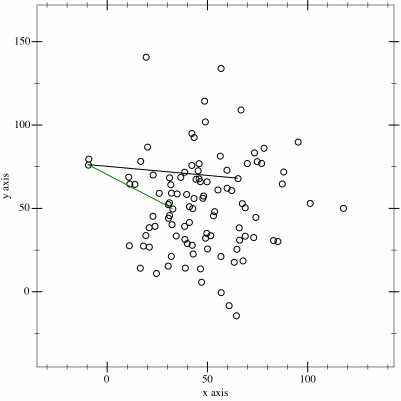By Maonus CC BY-SA 4.0, from Wikimedia Commons

To find the convex hull of the shadow, I’ve followed the Gift-wrapping algorithm straight from the pseudocode provided in the Wiki article.

One more thing, to determine whether a point is on the left of the line, I’ve used this StackOverflow article. The resulting code looked like this:

``````def get_2d_convex_hull(S):
def leftmost_point(S):
return min(S, key=lambda p: p)

def is_on_left(x, start, end):
# is x on the left from line from start to end?
return ((end - start)*(x - start) - (end - start)*(x - start)) > 0

P = []
point_on_hull = leftmost_point(S)
i = 0
while True:
P.append(point_on_hull)
endpoint = S
for j in range(len(S)):
if endpoint == point_on_hull or is_on_left(S[j], P[i], endpoint):
endpoint = S[j]
i += 1
point_on_hull = endpoint

if endpoint == P:
break

return P
``````

And to find the area of the convex hull, another StackOverflow article came to the rescue:

``````def get_convex_hull_area(S):
def area(p):
return 0.5 * abs(sum(x0*y1 - x1*y0
for ((x0, y0), (x1, y1)) in segments(p)))

def segments(p):
return zip(p, p[1:] + [p])

return area(S)
``````

Great! Now we can rotate the cube to a specific angle, and calculate what is the area of its shadow.

Putting 4.1. and 4.2. together we can calculate the area of cube’s shadow once it’s rotated to a specific angle:

``````def get_step(angle_alpha, angle_beta, angle_gamma):
faces = [(0.5, 0, 0), (0, 0.5, 0), (0, 0, 0.5)]
verteces = [
(0.5, 0.5, 0.5), (0.5, 0.5, -0.5),
(0.5, -0.5, 0.5), (0.5, -0.5, -0.5),
(-0.5, 0.5, 0.5), (-0.5, 0.5, -0.5),
(-0.5, -0.5, 0.5), (-0.5, -0.5, -0.5)
]

new_faces = rotate_matrix(faces, angle_alpha, angle_beta, angle_gamma)
new_verteces = rotate_matrix(verteces, angle_alpha, angle_beta, angle_gamma)

projected_verteces = project_3d_to_2d(new_verteces)

hull = get_2d_convex_hull(list(set(projected_verteces)))
area = get_convex_hull_area(hull)

return {
'faces': new_faces,
'area': area,
}
``````

We start with the initial cube, and then rotate both face and vertex vectors to the same angle. We’ll use vertex vectors for optimization, while face vectors will be necessary for the answer.

3D vertex vectors are converted to 2D ones by omitting the `Y` coordinate, and then that matrix is turned into a set to eliminate duplicate coordinates.

The function is named `get_step()`, as we will iteratively change all three angles by small amounts to find the right values such that the area is as close to the desired target as we can get.

#### 4.4. Function optimization

The goal is to find angles `alpha`, `beta` and `gamma`, so that `get_step(alpha, beta, gamma)` gives an answer close to the `target` value. So the smaller `abs(target - get_step(alpha, beta, gamma)` is - the better our answer is.

There are a number of algorithms to consider. I looked into Gradient descent I knew a bit about from Neural Network classes, but that didn’t work.

After some searching I found an implementation for Continuous hill climbing and Random Optimization. I went for a slightly modified version of Continuous hill climbing algorithm.

Upon implementation of the exact same hill climbing algorithm from the wiki article I found that it tended to always converge to `1.732050`, which seems to be the maximum area the shadow can cover, even though the target was different. So I had to modify scoring slightly.

The original hill climbing algorithm starts from `-infinity` and tries to climb up. Because our goal is to get to zero, I changed the algorithm to start from `+infinity`, and modified `best_score` evaluation to: `evaluate = lambda score: abs(score - target)`

The idea of the algorithm is fairly simple. In each iteration it tries to nudge a single variable in some direction. If doing so gives a better scoring answer than it had before - then it uses that newly discovered point for the next iteration, gradually inching closer and closer to the goal.

It can be implemented as follows:

``````def solve(target):
df = lambda alpha, beta, gamma: get_step(alpha, beta, gamma)['area']
evaluate = lambda score: abs(score - target)
alpha = 0
beta = 0
gamma = 0

PRECISION = 10e-10
current_point = [1, 1, 1]
step_size = [1, 1, 1]
acceleration = 12
candidate = [-acceleration, -1 / acceleration, 0, 1 / acceleration, acceleration]

while True:
before = df(*current_point)
for i in range(len(current_point)):
best = -1
best_score = math.inf

for j in range(5):
current_point[i] += step_size[i] * candidate[j]
temp = df(*current_point)
current_point[i] -= step_size[i] * candidate[j]
if evaluate(temp) < best_score:
best_score = evaluate(temp)
best = j

if candidate[best] == 0:
step_size[i] /= acceleration
else:
current_point[i] += step_size[i] * candidate[best]
step_size[i] *= candidate[best]

t = df(*current_point)
if abs(target - t) < PRECISION:
solution = get_step(*current_point)
return solution['faces']

return []
``````

And turns out, in this case it converges fairly quickly to the target `1.414213`:

``````Area               Error
1.4091240054698353 0.005088994530164648
1.4119704586438513 0.002242541356148653
1.4126303658805988 0.0015826341194011828
1.414195691609093 1.730839090696712e-05
1.4142002234618065 1.277653819342639e-05
1.4142116144140569 1.385585943092238e-06
1.414212563509047 4.3649095293751827e-07
1.4142130175986523 1.7598652313211005e-08
1.4142129999828152 1.7184698108962948e-11

[(0.16611577741917438, 0.47116771198777596, -0.020162729791066175), (-0.2584057051447727, 0.1088197672910437, 0.4139864125733548), (0.394502268740062, -0.12711902071258785, 0.27965821019955156)]
``````

Or to `1.732050`:

``````Area              Error
1.721904229213536 0.010145770786464059
1.723231518102203 0.008818481897796993
1.731250867053177 0.0007991329468231001
1.731673628707239 0.0003763712927611351
1.7316954153046518 0.0003545846953483256
1.7318965769362784 0.00015342306372168046
1.7319103991653426 0.00013960083465747175
1.7320214355685835 2.856443141663334e-05
1.7320272993244448 2.2700675555320515e-05
1.7320482657017473 1.7342982527868145e-06
1.7320496821790208 3.1782097931198905e-07
1.7320497962679902 2.037320099290696e-07
1.732049804975619 1.9502438108887077e-07
1.7320498998698852 1.0013011486620371e-07
1.7320499073756168 9.262438327439781e-08
1.7320499949575732 5.0424269204540906e-09
1.732050001429884 1.4298839889903547e-09
1.7320500000601848 6.018474607571989e-11

[(0.17916829614835567, 0.28883339349608833, 0.3667069571971998), (-0.22756542797063706, -0.28890843314528813, 0.3387416320000767), (0.40756925503260905, -0.2882831733887158, 0.02793052618723268)]
``````

This solution worked for both tests, succeeding in 2/2 tests and getting 32 points. Only 1158 contestants solved both correctly, I’m happy to be one of them.

### Final thoughts

Overall, it was fun. Not too happy about my performance with the first two problems, I think I could’ve gotten them if I was more attentive.

The last problem, just like in the Practice round, was tough, but satisfying to solve, and I’ve certainly learned a lot.

First is the value of knowing how such algorithms work and being able to implement them yourself. Sure, having a library take care of it is convenient, but one still needs to know what’s under the hood. Matrix operations, finding the convex hull and its area, function optimization were the big ones.

In the end I’ve solved all 4 problems, but got both test cases only for the last one (first: 1/2, second: 1/2, third: 1/2, fourth: 2/2). I got a total score of 55/100 and placed 5872/21300. Over 24 thousand people have participated in this round, which is way more than 4199 in the Practice round. Unexpected!

Considering that it took me about 10 hours for all 4 problems and that the first round’s duration is only 2 hours, I’m not sure I’ll participate in the next round. But, anyone who got more than 25 points will be invited to the next round, so we’ll see.

All in all I think it was a decent investment of a half of a weekend. And if you would like to crack your brain on some programming problems too, consider joining Google Code Jam next year, the event is organized yearly.

And if you have participated in the competition, how did you approach these problems?

• ## Google Code Jam 2018: Practice session results

If you’re not familiar with Google Code Jam, it’s a yearly competition organized by Google where contestants compete in solving programming problems. You are given a problem and some limitations, e.g. only a few hours to prepare solutions in later stages of the event, runtime and memory constraints for programs. It’s a fairly popular contest, bringing in thousands of participants from all over the world, with World Finals taking place in Toronto and the best contestant taking home a \$15,000 grand prize, fame and glory.

In the practice session of 2018 there were 4 problems and 2 days were given to solve them. You can find the full list of problems and their descriptions HERE.

### 1. Number Guessing

But in short, the judge program comes up with a secret number in a given range and allows you to make no more than a specific number of guesses. Your program has to guess what the number is, and after each guess the judge will respond with “TOO_BIG”, “TOO_SMALL” or “CORRECT”. If your program fails to guess the number correctly, the judge will respond with “WRONG_ANSWER” and will stop replying.This immediately sounds like binary search, doesn’t it? You have to guess a number in 1-dimensional space, and the only feedback judge provies is if we guessed to high or too low. So the best we can do is to always guess in the middle of the available space, discarding the half where we know our target is not in. Based on the image example above, it would take 4 guesses to find the right answer using this strategy: binary search has an average performance of O(log(n)).

My first stumble was in picking the right midpoint. Initial one was the average of lower and upper bounds `midpoint = (lower_bound + upper_bound) // 2`. Integer division is there to avoid producing a decimal number (e.g. `(1 + 4) / 2 == 2.5`, but `(1 + 4) // 2 == 2`). This passed the `testing_tool.py`, but failed hidden tests, failing to find the answer in required number of guesses. Wiki article also suggests using `floor` of `(lower_bound + upper_bound) / 2`, but taking `ceil` instead passed the tests: `midpoint = (lower_bound + upper_bound + 1) // 2`.

The second stumble was in not terminating the program as specified when the judge responded with `WRONG_ANSWER`. It wasn’t quite clear whether on the last answer it would respond with `TOO_BIG` and then `WRONG_ANSWER`, or just `WRONG_ANSWER`, so I covered for both, yielding this code:

``````def run():
for case in range(test_cases):
case += 1

guessed_right = False

for guess in range(guesses):
midpoint = (lower_bound + upper_bound + 1) // 2
write_guess(midpoint)

upper_bound = middle
lower_bound = middle
guessed_right = True
break
exit()

if guessed_right == False:
exit()
``````

I skipped definitions for `read_int()`, `read_ints()`, `read_answer()`, `write_guess()` - they’re basic `stdin` and `stdout` operations.

### 2. Senate evacuation

In short, there are a number of political parties in senate with some number of senators in each party. We want to evacuate all senators in such a way that neither party has the majority at any step (>50% of senators still in senate), while we can evacuate only one or two senators at a time.

Or to rephrase the problem slightly differently: we have a list of integers, we can subtract 1 or 2 at a time from any member in such a way that neither member is bigger than 50% of sum of all members. Let’s start with checking the current state for validity and encode this rephrased version in code:

``````def is_valid(senators):
total_senators = sum(senators)
limit = total_senators / 2
return all(map(lambda p: p <= limit, senators))
``````

For the strategy itself, initial hunch suggests to attack parties with most senators first. We will always be able to evacuate at least one senator. As for the second one, after selecting the first candidate we can check again which party has most members. If after removing it the state is still legal (using `is_valid()`) - then we can take two senators. If not - we have to take just one. We just have to take into account a case when there are no senators left at all (`[0, 0, ..., 0]`), or just one (`[1, 0, 0, ..., 0]`). In code this idea can be written as:

``````def solve(parties, senators):
solution = []
most_senators = max(senators)
while most_senators > 0:
first_target = senators.index(most_senators)
senators[first_target] -= 1

second_largest_party = max(senators)
second_target = senators.index(second_largest_party)
senators[second_target] -= 1

if second_largest_party == 0 or not is_valid(senators):
# Can take only one senator, must put second one back
solution.append(alphabet[first_target])
senators[second_target] += 1
else:
# Can take both senators
solution.append(alphabet[first_target] + alphabet[second_target])

most_senators = max(senators)
return solution
``````

And to transform political party numbers back into alphabetical party names: `alphabet = dict(enumerate('ABCDEFGHIJKLMNOPQRSTUVWXYZ'))`.

With final `run()` written as:

``````def run():
for case in range(test_cases):
case += 1
solution = solve(N, senators)

write_answer('Case #%d: %s' % (case, ' '.join(solution)))
``````

### 3. Steed 2: Cruise Control

In short, on a one-way road there are a number of horses with some initial positions and all travelling in the same direction at some individual speeds. However, these horses move in a special way in that they cannot pass each other. So if one catches up to another, they’ll both continue travelling at the slower one’s speed. Our hero wants to travel to a specific point on the same road and has to abide same rules of not passing another horse. The hero can travel at any speed, but wants to maintain a constant speed throughout the full travel without having to speed up or slow down. What is the maximum speed the hero can choose?

The first hunch was to try and calculate for how long each horse will travel at what speed, and try to deduce hero’s target speed from that. But the problem mentions that it may use up to 1000 horses. At least we can imagine describing a situation where we put fastest horses at the start, and slowest ones near the end, and faster horses gradually running into slower ones. It quickly becomes a complex mess.

The second idea was to compute how long it will take for each horse to get to hero’s destination, but do it from right to left. Right to left, because horses to the right will always be limiting factors - horses to the left can’t get to the destination faster than the horse to the right, they’d eventually catch up and will have to slow down, so their journey will take at least as long as the horse’s to the right. And turns out that this approach allows for fairly straightforward linear computation.

So for each horse we compute how long it would take them to cover the remaining distance from their starting point to the destination. If we take the `max()` of these durations, that will give us our hero’s travel duration. From there we can find the average speed with `average_speed = distance / duration`.

``````def solve(distance, total_horses, horses):
longest_duration = max(map(lambda h: (distance - h) / h, horses.values()))
return distance / longest_duration

def run():
for case in range(test_cases):
case += 1
horses = {}
for i in range(total_horses):
horses[i] = [initial, max_speed]
# print([distance, total_horses, horses])
solution = solve(distance, total_horses, horses)

write_answer('Case #%d: %.7f' % (case, solution))
``````

### 4. Bathroom Stalls

In short, there are N+2 bathroom stalls, with first and last one already occupied. A number of people will come in one after another, selecting a stall and never leaving. People will select a stall that is furthest from its closest neighbors. The goal is to find out, when the last person takes a stall, what’s the largest and lowest number of free stalls next to the side of it.

This one was rough, taking about 4 hours to figure out, but in the end the solution was mathematical and fairly elegant, at least in my eyes.

The initial idea would be to maybe try and write a program that puts people in the appropriate stalls sequentially one by one. But the problem arises from constraints: the number of stalls can be as large as 10^18, and number of people can be equal to the number of stalls.

How would such data structure be represented? If we represented each stall as 1 byte, that’s still 10^18 bytes - exabyte, for comparison a gigabyte is about 10^9, so there’s no way we’re storing this much information, in memory or on disk. northeastern.edu suggests that in 2013 total amount of data in the world was 4.4 zettabytes, or 4400 exabytes. We certainly won’t dedicate `0.2%` of world’s storage to solve this problem. And If each stall was represented as a bit, divide the numbers by 8, but they’re still gargantuan and unmanageable numbers.

Especially note that the execution time limit is 30 seconds. Which suggests there has to be a better way.

The next idea was that maybe it could be related to fractals - similar repeating patterns at different scales. E.g. Here’s how 7 stalls would be filled up:

``````1|2|3|4|5|6|7  1|2|3|4|5|6|7
-------------  -------------
_|_|_|1|_|_|_  _|_|_|1|_|_|_
_|2|_|_|_|_|_  _|2|_|1|_|_|_
_|_|_|_|_|3|_  _|2|_|1|_|3|_
4|_|_|_|_|_|_  4|2|_|1|_|3|_
_|_|5|_|_|_|_  4|2|5|1|_|3|_
_|_|_|_|6|_|_  4|2|5|1|6|3|_
_|_|_|_|_|_|7  4|2|5|1|6|3|7
``````

Maybe the same pattern that governs selection of one could be repeated on its left branch to position 2, resulting maybe in a tree of sorts. But that still does not suggest a way how to determine number of free stalls on each position’s side. So the third option was to brute force and write down free stall numbers for each N.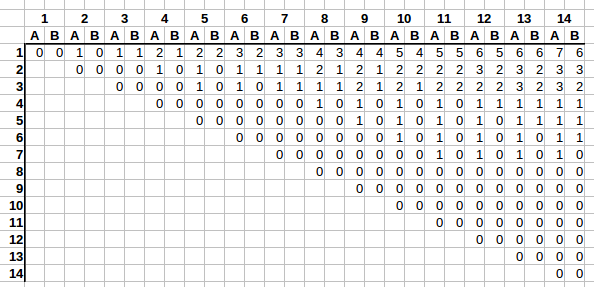In the graph the columns denote `N` - how many stalls there are. Rows denote how many people have already selected a stall. And (stalls, people) combination denotes `A` - the largest number of stalls available next to last person’s stall, and `B` - the lowest number of stalls next to the last selected stall.

Right away we see an interesting trend. E.g. take a look at the first row. As columns grow from left to right, first `A` increases by one, then `B` catches up on the next column. And it repeats.

On the second row it’s very similar. First `A` increases by one, then `B` catches up, but this rate of change is slower, only every two columns as opposed to with every one.

It’s getting difficult to follow, as we’re running out of columns to clearly see the trend, but on the fourth row the rate of change is even slower, with `A` and `B` incrementing only once every 4 columns.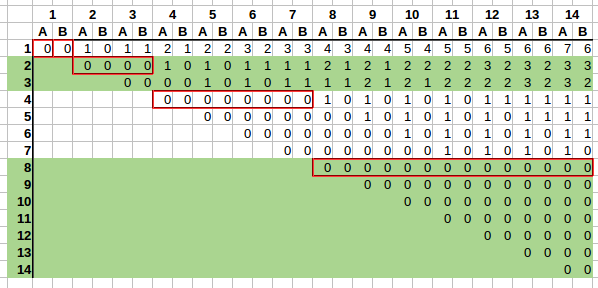It would be a bit clearer if we had 16 rows and 16 columns, but this suffices to spot the trend.

We can see that rate of change also changes based on the row, and such rates of change can be grouped based on powers of two:

• `2^0 = 1` - one row will change on every column;
• `2^1 = 2` - there are 2 rows in the group, so `A` and `B` wil change every 2 columns on rows `2` and `3`;
• `2^2 = 2` - there are 4 rows in the group, `A` and `B` will change every `4` columns on rows `4`-`7`;
• `2^3 = 8` - there are 8 rows in the group, `A` and `B` will change every `8` columns on rows `8`-`15`;

And so on. We have found a pattern. Now how to calculate `A` and `B` if we are given the row and column number?

#### Rate of change

First we need to find every how many columns `A` and `B` changes based on the row. Since groups grow as powers of two, we could take `floor(log2(row))` to find in which group a row is. E.g. `floor(log2(9)) = 3`, which means we are in the fourth group (indexed from 0, see list above). If we raise the base 2 back to the power of `3` - this gives us rate of change `8`, which is what we would expect as per our pattern we’ve seen above.

So the derived formula for rate of change seems to be `rate_of_change = lambda row: 2 ** math.floor(math.log2(row))`

#### How many changes already occurred

Next we need to find out how many changes have occurred based on the column number. We can see that `A` and `B` always change in the following pattern:

`0 0 -> 1 0 -> 1 1 -> 2 1 -> 2 2 -> 3 2 -> 3 3 -> ... -> x+1 x -> x+1 x+1 -> ...`

So based on rate of change we can count how many changes have already occurred given a row and a column.

Taking a look at the stall positioning table again, for any row number, on which column does the row start? We see that the row starts at the column number equal to the row number. E.g. row 4 starts at 4th column, row 9 starts at 9th column. So if we check how many `rate_of_change` columns fit in this space - that will tell us how many changes have already occurred.

E.g. for row=3, column=6, the rate of change is `2 ** math.floor(math.log2(3)) == 2`. Row 3 starts at column `3`, and we’re interested in column `6`. `6-3` leaves a space of 3 for changes to occur in. If we divide that by `rate_of_change = 2` => `(6 - 3) / 2 = 1.5`. So a full one change has already occurred, going `0 0 -> 1 0`. If you check the table again, we see that this is exactly right.

So the derived formula seems to be `number_of_changes_done = lambda column, row: math.floor((column - row) / rate_of_change(row))`

#### Putting it all together: calculating A and B

Now we can calculate `A` and `B`.

If we take a look at the first line, we see that `A` number seems to be equal to about half of the number of changes for a specific column. E.g. on row=1 column=3, the result we are aiming for is `A=1, B=1`, and a total of 2 changes have already occurred. Or on row=1 column=10, the result we are aiming for is `A=5, B=4`, and a total of 9 changes have already occurred.

And `B` tends to also be about half of the number of changes for a specific column, but always either equal to `A`, or one less than `A`.

So my idea was to use `ceil` and `floor`, setting `A = math.ceil(number_of_changes_done / 2)`, and `B = number_of_changes_done - A`, and this seems to work.

E.g. row=1 column=3: `number_of_changes_done = 2`, `A = math.ceil(2 / 2) => math.ceil(1) => 1`, `B = 2 - 1 => B = 1`. Or row=6, column=14: `number_of_changes_done = 2`, `A = math.ceil(2 / 2) => math.ceil(1) => 1`, `B = 2 - 1 => B = 1`. Or row=2, column=11: `number_of_changes_done = 4`, `A = math.ceil(4 / 2) => math.ceil(2) => 2`, `B = 4 - 2 => B = 2`.

The formulas seem to check out!

#### Final code

``````def get_change_rate_on_row(K):
# Every how many records do values change on row K
return 2 ** math.floor(math.log2(K))

def number_of_changes_done(N, K):
return math.floor((N - K) / get_change_rate_on_row(K))

def solve(N, K):
change_rate = get_change_rate_on_row(K)

total_changes = number_of_changes_done(N, K)

y = math.ceil(total_changes / 2)
z = total_changes - y

return [str(y), str(z)]

def run():
for case in range(test_cases):
case += 1

solution = solve(stalls, people)

# print(case, solution)
write_answer('Case #%d: %s' % (case, ' '.join(solution)))
``````

All this work for a straight forward calculation! But there we go, we have calculated values `A` and `B` for any given row and column in a fraction of a second and without using 0.02% of world’s data storage capacity.

### Final thoughts

The final result ended up being:

• Place: 661/4199
• Score: 60
• Problem 1: 2/2
• Problem 2: 2/2
• Problem 3: 2/2
• Problem 4: 2/3. Last test with 1 <= N <= 10^18 failed with “Wrong answer”. Unfortunately data is not published, so I can’t check what happened, especially at that scale.

But overall it’s been a fun brain teaser. The last problem was especially challenging, but that made it so much more satisfying to crack.

The first problem seemed like a clear approach for binary search, but I was unable to think of any metaphors or similar problems for the other three. I managed to muddle through them, but seeing how quickly other contestands were able to breeze through these problems, that makes me think that there are other relatable problems or approaches one could use here. Have you solved these problems? What approach did you take? I’d be curious to learn more.

And if you haven’t tried Google Code Jam or similar competitions before - give it a go some time. They can twist your brain unlike daily CRUD apps, making us more capable of solving more complex problems in the end.